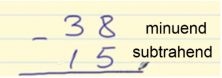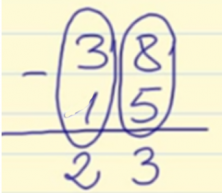Accelerate your child’s learning
Smartick is a fun way to learn math!Jun08

# Learn How to Subtract without Regrouping

Hello and welcome back! Today we are going to learn how to subtract without regrouping. Are you ready?

### Subtract Without Regrouping

We’re going to follow a few steps. If you follow them well, you’ll learn how to subtract without regrouping in no time:

1. You need to place the subtrahend below the minuend so that the ones-place numbers fall in the same column.
2. Now you have to subtract each column separately and in order, starting with the ones-place column.
3. The last step is to place the answer of the subtractions below each one of the columns, and remember: always put it in order!

It’s easy, right? Let’s practice with an example:We need to write down 38 and below it, 15, making sure that the 5 is just below the 8.We start off by subtracting the ones-place column: 8 – 5 = 3, and we write the 3 under the same column.

Now, let’s solve the 10s-place column: 3 – 1 = 2, and we write the 2 below the column.

As you can see above, the answer is 23.

That’s all for today, I hope you learned how to subtract without regrouping.

In the following link, you’ll learn more about regrouping:

If you want to keep learning more Elementary Math, try Smartick for free.

Fun is our brain’s favorite way of learning
Diane Ackerman
Smartick is a fun way to learn math
• 15 fun minutes a day
• Millions of students since 2009